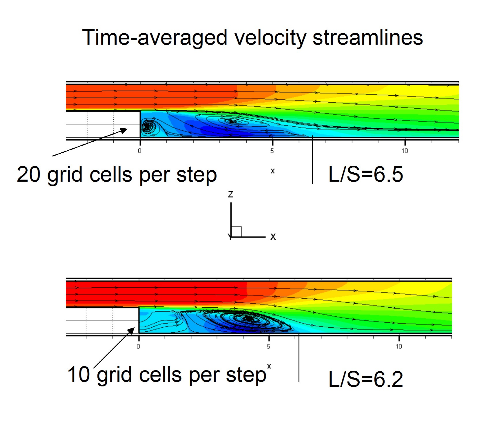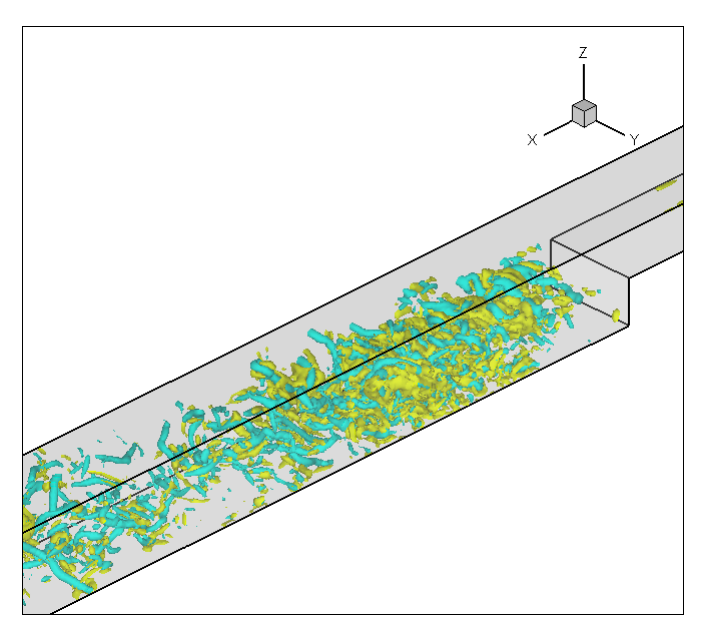CABARET Demonstration

To demonstrate the method, Figureshows the normalised x direction velocity component of the flow field at 40000 time steps the evolving solution after 10000 time steps for the 3D backward facing step test case. Here, the boundary conditions are for laminar flow, with Reynolds number=5000 and Mach number=0.1. The grid size is 10 points per the step height giving a total grid size of 800000 points. Time-averaged velocity fields shown for this mesh resolution and for a 20 cells per step height give the correct length for the recirculation zone around the step as shown in Figure. The Vorticity (Q-norm) iso-surfaces for the grid density of 20 cells per step are shown in Figure.Phil Ridley 2011-02-01